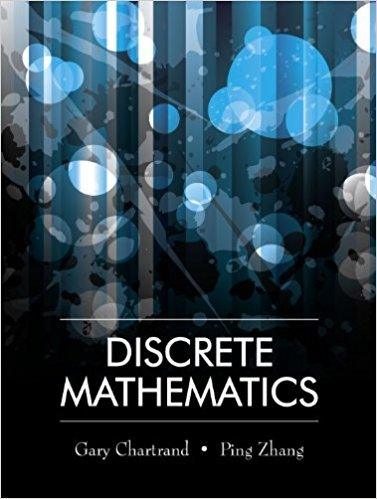×
×

# Let x be a real number. Prove that if (x2 1)2 = 0, then x4 x2 = 0ISBN: 9781577667308 385

## Solution for problem 5 Chapter 3

Discrete Mathematics | 1st Edition

• Textbook Solutions
• 2901 Step-by-step solutions solved by professors and subject experts
• Get 24/7 help from StudySoup virtual teaching assistantsDiscrete Mathematics | 1st Edition

4 5 1 346 Reviews
14
1
Problem 5

Let x be a real number. Prove that if (x2 1)2 = 0, then x4 x2 = 0.

Step-by-Step Solution:
Step 1 of 3
Step 2 of 3

Step 3 of 3

##### ISBN: 9781577667308

Unlock Textbook Solution